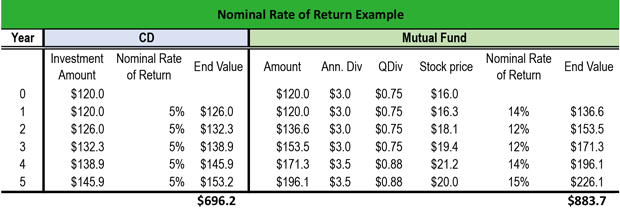# What is the Nominal Rate of Return?

Definition: Nominal rate of return represents the revenue of an investment before considering tax and inflation expenses.

## What Does Nominal Rate of Return Mean?

What is the definition of nominal rate of return? The rule of thumb in finance is that the value of money today is higher that the value of money in the future because of interest rates and inflation. When calculating investment returns, analysts determine the difference between the nominal rate and the real return, which adjusts to the current purchasing power. If the expected inflation rate is high, investors expect a higher nominal rate.

However, in some cases, the nominal rate is misleading. For example, if an investor holds a corporate bond and a municipal bond with a nominal value of \$1,000 and an expected nominal rate 5%, one would assume that the bonds are of equal value. However, corporate bonds are taxed at 30%, whereas munis are tax exempt. Therefore, their real rate of return is completely different.

Let’s look at an example.

## Example

Jamal buys a certificate of deposit for \$120 at an annual interest rate of 5%. This means that Jamal will earn \$120 x 5% = \$6 per year. If he invests \$120 in a mutual fund at an annual interest rate of 5%, he will earn \$6 per year as well. However, the mutual fund pays an annual dividend of \$3. So, the nominal rate is different for the two investments.

Buying the CD for 5 years, Jamal will earn 5% annually; so, at the end of the investment period in year 5, he will have \$696.2 However, by investing in the mutual fund, he will have \$883.7.Since the fund pays an annual dividend of \$3, the quarterly dividend is 0.75. To find that nominal rate, Jamal multiplies the annual dividend by the quarterly dividend over the stock price:

1. Year 1: 3 x (0.75/16.3) = 14%
2. Year 2: 3 x (0.75/18.1) = 12%
3. Year 3: 3 x (0.75/19.4) = 12%
4. Year 4: 3.5 x (0.88/21.2) = 14%
5. Year 5: 3.5 x (0.88/20.0) = 15%

Notice that in the years 4 and 5, the annual dividend increase to \$3.5, so the quarterly dividend increases to \$0.88.

## Summary Definition

Define Nominal Rate of Return: Nominal rate means the earnings from an investment before taking into consideration its tax and inflation consequences.